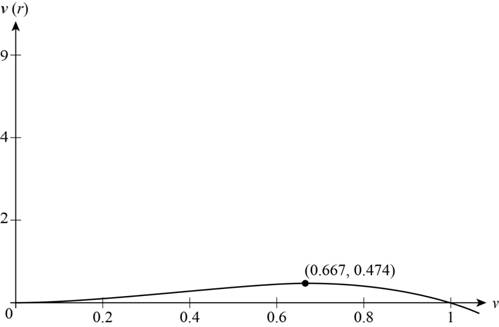# The value of r for which v is maximum.### Precalculus: Mathematics for Calcu...

6th Edition
Stewart + 5 others
Publisher: Cengage Learning
ISBN: 9780840068071### Precalculus: Mathematics for Calcu...

6th Edition
Stewart + 5 others
Publisher: Cengage Learning
ISBN: 9780840068071

#### Solutions

Chapter 2.3, Problem 55E
To determine

## The value of r for which v is maximum.

Expert Solution

The value of r is 0.6667 for which the value of v is maximum.

### Explanation of Solution

A function of velocity v of the airstream through trachea of radius r is given by

v(r)=3.2(1r)r2 and the value of r is 12r1 .

Use graph calculator to draw thee graph of the function v(r)=3.2(1r)r2 as shown below.Figure (1)

Locate the point of local maximum in the above graph to find the value of r for which v is maximum.

The value v(a) is local maximum value if v(a)v(x) when a is near x.

From Figure (1), it is noticed that there is a local maximum between x=0.5 and x=1 .

Point (0.6667,0.474) is the highest point on the graph. This point is the point of local maximum.

The value 0.474 is the local maximum value at x=0.6667 .

Therefore, the value of r is 0.6667 for which the value of v is maximum.

### Have a homework question?

Subscribe to bartleby learn! Ask subject matter experts 30 homework questions each month. Plus, you’ll have access to millions of step-by-step textbook answers!